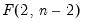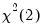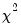cellipse
Confidence ellipses for coefficient restrictions.
The cellipse view displays confidence ellipses for pairs of coefficient restrictions for an estimation object.
Syntax
system_name.cellipse(options) restrictions
Enter the object name, followed by a period, and the keyword cellipse. This should be followed by a list of the coefficient restrictions. Joint (multiple) coefficient restrictions should be separated by commas.
Options

 ind=arg Specifies whether and how to draw the individual coefficient intervals. The default is “ind=line” which plots the individual coefficient intervals as dashed lines. “ind=none” does not plot the individual intervals, while “ind=shade” plots the individual intervals as a shaded rectangle. size=number (default=0.95) Set the size (level) of the confidence ellipse. You may specify more than one size by specifying a space separated list enclosed in double quotes. dist=arg Select the distribution to use for the critical value associated with the ellipse size. The default depends on estimation object and method. If the parameter estimates are least-squares based, thedistribution is used; if the parameter estimates are likelihood based, thedistribution will be employed. “dist=f” forces use of the F-distribution, while “dist=c” uses thedistribution. prompt Force the dialog to appear from within a program. p Print the graph.
Examples
The two commands:
sys1.cellipse c(1), c(2), c(3)
sys1.cellipse c(1)=0, c(2)=0, c(3)=0
both display a graph showing the 0.95-confidence ellipse for C(1) and C(2), C(1) and C(3), and C(2) and C(3).
sys1.cellipse(dist=c,size="0.9 0.7 0.5") c(1), c(2)
displays multiple confidence ellipses (contours) for C(1) and C(2).
Cross-references
See “Confidence Intervals and Confidence Ellipses” for discussion.